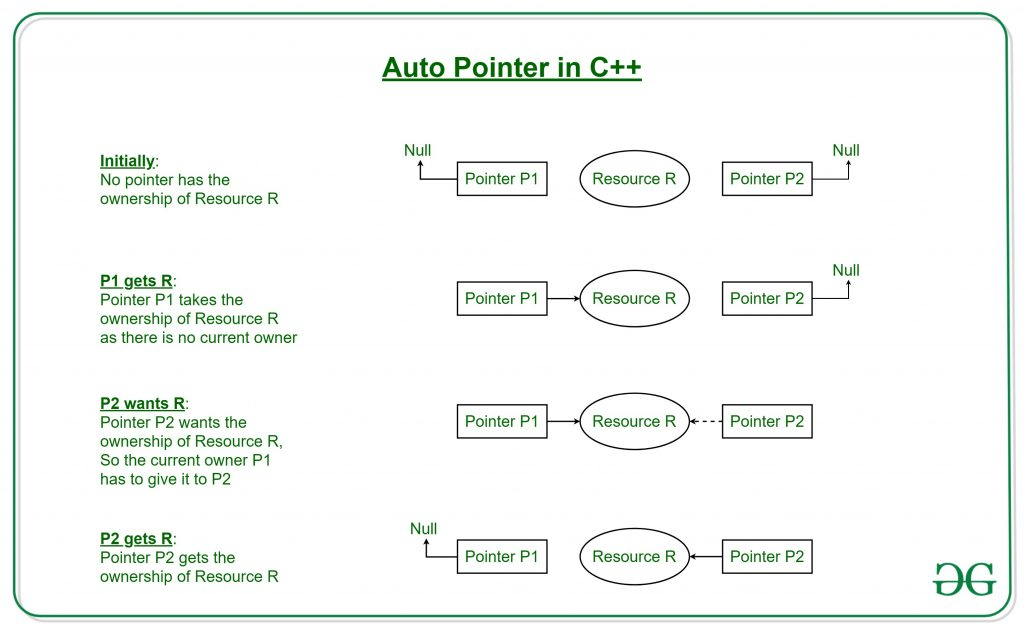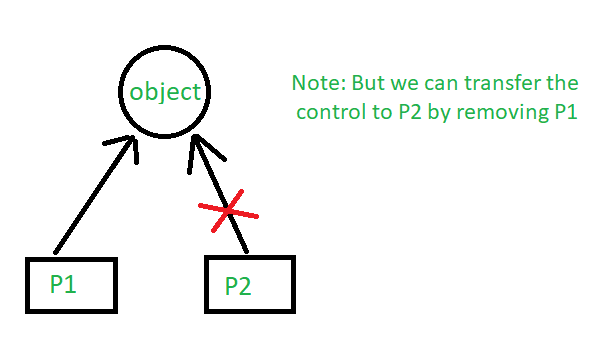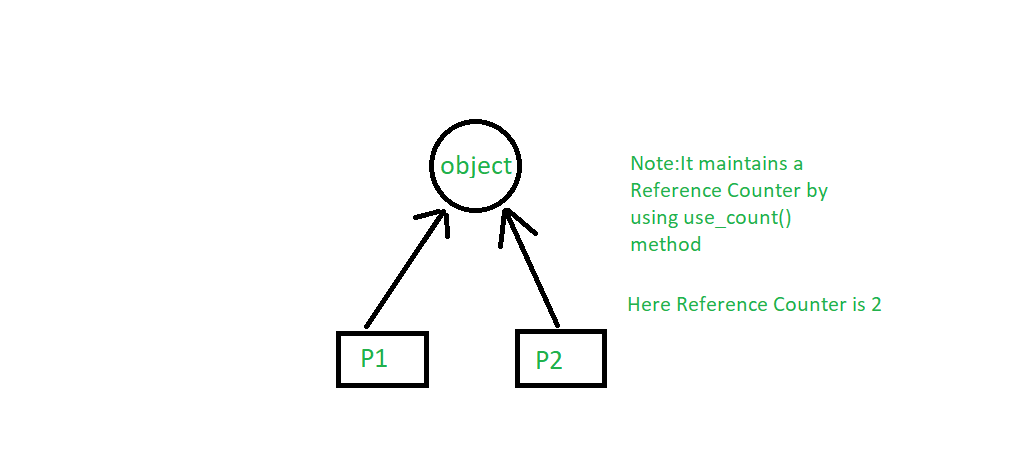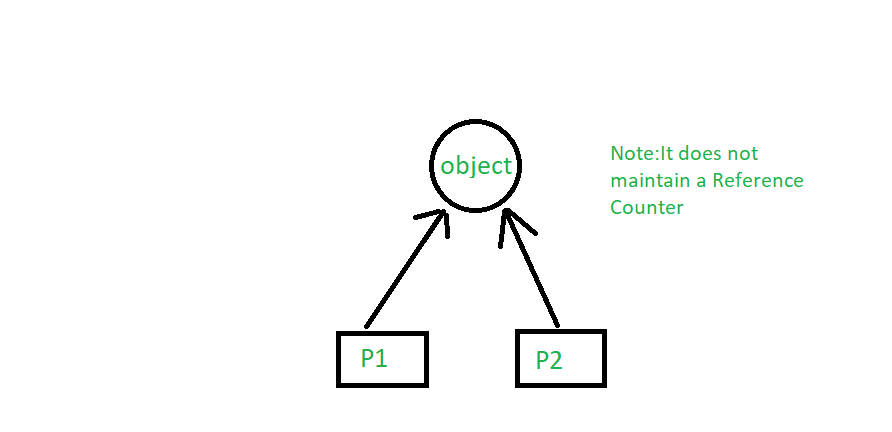GFG App
Open AppBrowser
Continue

# Smart Pointers in C++

Prerequisite: Pointers in C++

Pointers are used for accessing the resources which are external to the program – like heap memory. So, for accessing the heap memory (if anything is created inside heap memory), pointers are used. When accessing any external resource we just use a copy of the resource. If we make any changes to it, we just change it in the copied version. But, if we use a pointer to the resource, we’ll be able to change the original resource.

## Problems with Normal Pointers

Some Issues with normal pointers in C++ are as follows:

• Memory Leaks: This occurs when memory is repeatedly allocated by a program but never freed. This leads to excessive memory consumption and eventually leads to a system crash.
• Dangling Pointers: A dangling pointer is a pointer that occurs at the time when the object is de-allocated from memory without modifying the value of the pointer.
• Wild Pointers: Wild pointers are pointers that are declared and allocated memory but the pointer is never initialized to point to any valid object or address.
• Data Inconsistency: Data inconsistency occurs when some data is stored in memory but is not updated in a consistent manner.
• Buffer Overflow: When a pointer is used to write data to a memory address that is outside of the allocated memory block. This leads to the corruption of data which can be exploited by malicious attackers.

Example:

## C++

 `// C++ program to demonstrate working of a Pointers` `#include ` `using` `namespace` `std;`   `class` `Rectangle {` `private``:` `    ``int` `length;` `    ``int` `breadth;` `};`   `void` `fun()` `{` `    ``// By taking a pointer p and` `    ``// dynamically creating object` `    ``// of class rectangle` `    ``Rectangle* p = ``new` `Rectangle();` `}`   `int` `main()` `{` `    ``// Infinite Loop` `    ``while` `(1) {` `        ``fun();` `    ``}` `}`

Output:

`Memory limit exceeded`

Explanation: In function fun, it creates a pointer that is pointing to the Rectangle object. The object Rectangle contains two integers, length, and breadth. When the function fun ends, p will be destroyed as it is a local variable. But, the memory it consumed won’t be deallocated because we forgot to use delete p; at the end of the function. That means the memory won’t be free to be used by other resources. But, we don’t need the variable anymore, we need the memory.

In function main, fun is called in an infinite loop. That means it’ll keep creating p. It’ll allocate more and more memory but won’t free them as we didn’t deallocate it. The memory that’s wasted can’t be used again. Which is a memory leak. The entire heap memory may become useless for this reason.

## Smart Pointers

As we’ve known unconsciously not deallocating a pointer causes a memory leak that may lead to a crash of the program. Languages Java, C# has Garbage Collection Mechanisms to smartly deallocate unused memory to be used again. The programmer doesn’t have to worry about any memory leaks. C++ comes up with its own mechanism that’s Smart Pointer. When the object is destroyed it frees the memory as well. So, we don’t need to delete it as Smart Pointer does will handle it.

A Smart Pointer is a wrapper class over a pointer with an operator like * and -> overloaded. The objects of the smart pointer class look like normal pointers. But, unlike Normal Pointers it can deallocate and free destroyed object memory.

The idea is to take a class with a pointer, destructor, and overloaded operators like * and ->. Since the destructor is automatically called when an object goes out of scope, the dynamically allocated memory would automatically be deleted (or the reference count can be decremented).

Example:

## C++

 `// C++ program to demonstrate the working of Smart Pointer` `#include ` `using` `namespace` `std;`   `class` `SmartPtr {` `    ``int``* ptr; ``// Actual pointer` `public``:` `    ``// Constructor: Refer https:// www.geeksforgeeks.org/g-fact-93/` `    ``// for use of explicit keyword` `    ``explicit` `SmartPtr(``int``* p = NULL) { ptr = p; }`   `    ``// Destructor` `    ``~SmartPtr() { ``delete` `(ptr); }`   `    ``// Overloading dereferencing operator` `    ``int``& operator*() { ``return` `*ptr; }` `};`   `int` `main()` `{` `    ``SmartPtr ptr(``new` `int``());` `    ``*ptr = 20;` `    ``cout << *ptr;`   `    ``// We don't need to call delete ptr: when the object` `    ``// ptr goes out of scope, the destructor for it is automatically` `    ``// called and destructor does delete ptr.`   `    ``return` `0;` `}`

Output

`20`

### Difference Between Pointers and Smart Pointers

Note: This only works for int. So, we’ll have to create Smart Pointer for every object? No, there’s a solution, Template. In the code below as you can see T can be of any type.

Example:

## C++

 `// C++ program to demonstrate the working of Template and` `// overcome the issues which we are having with pointers` `#include ` `using` `namespace` `std;`   `// A generic smart pointer class` `template` `<``class` `T> ``class` `SmartPtr {` `    ``T* ptr; ``// Actual pointer` `public``:` `    ``// Constructor` `    ``explicit` `SmartPtr(T* p = NULL) { ptr = p; }`   `    ``// Destructor` `    ``~SmartPtr() { ``delete` `(ptr); }`   `    ``// Overloading dereferencing operator` `    ``T& operator*() { ``return` `*ptr; }`   `    ``// Overloading arrow operator so that` `    ``// members of T can be accessed` `    ``// like a pointer (useful if T represents` `    ``// a class or struct or union type)` `    ``T* operator->() { ``return` `ptr; }` `};`   `int` `main()` `{` `    ``SmartPtr<``int``> ptr(``new` `int``());` `    ``*ptr = 20;` `    ``cout << *ptr;` `    ``return` `0;` `}`

Output:

`20`

Note: Smart pointers are also useful in the management of resources, such as file handles or network sockets.

## Types of Smart Pointers

C++ libraries provide implementations of smart pointers in the following types:

• auto_ptr
• unique_ptr
• shared_ptr
• weak_ptr

## auto_ptr

Using auto_ptr, you can manage objects obtained from new expressions and delete them when auto_ptr itself is destroyed. When an object is described through auto_ptr it stores a pointer to a single allocated object.

Note: This class template is deprecated as of C++11. unique_ptr is a new facility with a similar functionality, but with improved security.## unique_ptr

unique_ptr stores one pointer only. We can assign a different object by removing the current object from the pointer.Example:

## C++

 `// C++ program to demonstrate the working of unique_ptr` `// Here we are showing the unique_pointer is pointing to P1.` `// But, then we remove P1 and assign P2 so the pointer now` `// points to P2.`   `#include ` `using` `namespace` `std;` `// Dynamic Memory management library` `#include `   `class` `Rectangle {` `    ``int` `length;` `    ``int` `breadth;`   `public``:` `    ``Rectangle(``int` `l, ``int` `b)` `    ``{` `        ``length = l;` `        ``breadth = b;` `    ``}`   `    ``int` `area() { ``return` `length * breadth; }` `};`   `int` `main()` `{` `// --\/ Smart Pointer` `    ``unique_ptr P1(``new` `Rectangle(10, 5));` `    ``cout << P1->area() << endl; ``// This'll print 50`   `    ``// unique_ptr P2(P1);` `    ``unique_ptr P2;` `    ``P2 = move(P1);`   `    ``// This'll print 50` `    ``cout << P2->area() << endl;`   `    ``// cout<area()<

Output

```50
50```

## shared_ptr

By using shared_ptr more than one pointer can point to this one object at a time and it’ll maintain a Reference Counter using the use_count() method.## C++

 `// C++ program to demonstrate the working of shared_ptr` `// Here both smart pointer P1 and P2 are pointing to the` `// object Addition to which they both maintain a reference` `// of the object` `#include ` `using` `namespace` `std;` `// Dynamic Memory management library` `#include `   `class` `Rectangle {` `    ``int` `length;` `    ``int` `breadth;`   `public``:` `    ``Rectangle(``int` `l, ``int` `b)` `    ``{` `        ``length = l;` `        ``breadth = b;` `    ``}`   `    ``int` `area() { ``return` `length * breadth; }` `};`   `int` `main()` `{` `    ``//---\/ Smart Pointer` `    ``shared_ptr P1(``new` `Rectangle(10, 5));` `    ``// This'll print 50` `    ``cout << P1->area() << endl;`   `    ``shared_ptr P2;` `    ``P2 = P1;`   `    ``// This'll print 50` `    ``cout << P2->area() << endl;`   `    ``// This'll now not give an error,` `    ``cout << P1->area() << endl;`   `    ``// This'll also print 50 now` `    ``// This'll print 2 as Reference Counter is 2` `    ``cout << P1.use_count() << endl;` `    ``return` `0;` `}`

Output

```50
50
50
2```

## weak_ptr

Weak_ptr is a smart pointer that holds a non-owning reference to an object. It’s much more similar to shared_ptr except it’ll not maintain a Reference Counter. In this case, a pointer will not have a stronghold on the object. The reason is if suppose pointers are holding the object and requesting for other objects then they may form a Deadlock.## C++

 `// C++ program to demonstrate the working of weak_ptr` `// Here both smart pointer P1 and P2 are pointing to the` `// object Addition to which they both does not maintain` `// a reference of the object` `#include ` `using` `namespace` `std;` `// Dynamic Memory management library` `#include `   `class` `Rectangle {` `    ``int` `length;` `    ``int` `breadth;`   `public``:` `    ``Rectangle(``int` `l, ``int` `b)` `    ``{` `        ``length = l;` `        ``breadth = b;` `    ``}`   `    ``int` `area() { ``return` `length * breadth; }` `};`   `int` `main()` `{` `    ``//---\/ Smart Pointer` `    ``shared_ptr P1(``new` `Rectangle(10, 5));`   `    ``// This'll print 50` `    ``cout << P1->area() << endl;`   `    ``auto` `P2 = P1;` `    ``// P2 = P1;`   `    ``// This'll print 50` `    ``cout << P2->area() << endl;`   `    ``// This'll now not give an error,` `    ``cout << P1->area() << endl;`   `    ``// This'll also print 50 now` `    ``// This'll print 2 as Reference Counter is 2` `    ``cout << P1.use_count() << endl;` `    ``return` `0;` `}`

Output

```50
50
50
2```

C++ libraries provide implementations of smart pointers in the form of auto_ptr, unique_ptr, shared_ptr, and weak_ptr

My Personal Notes arrow_drop_up